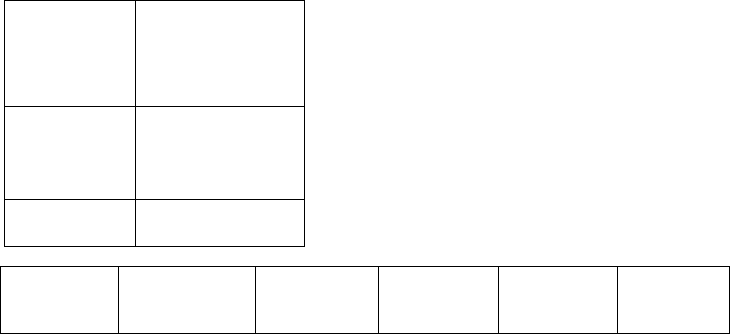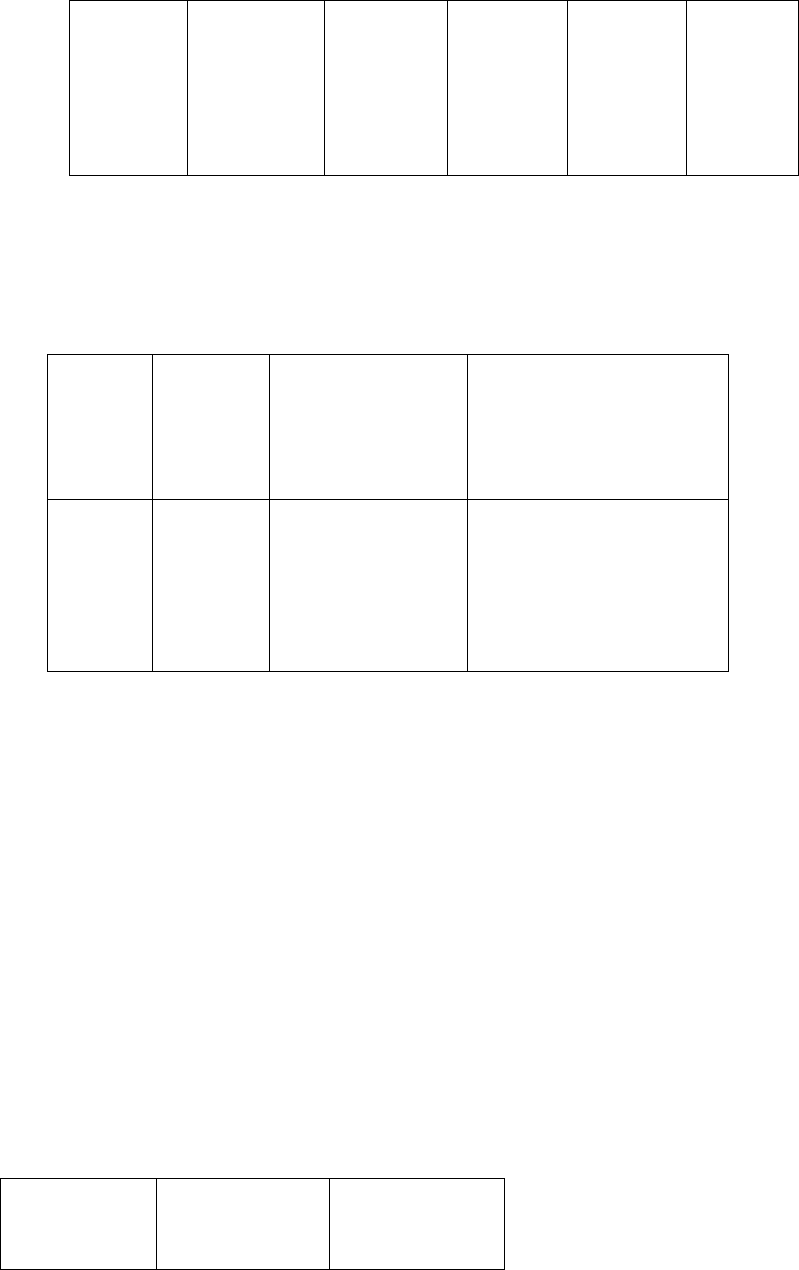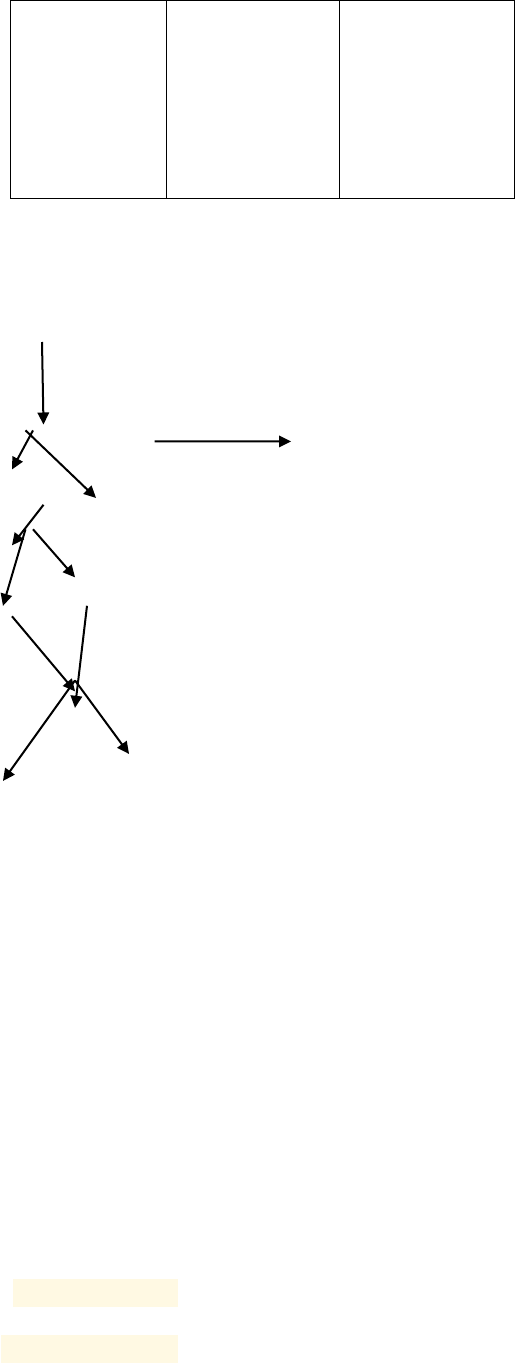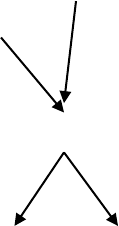Logic Maths | EssayIvy.com

# Logic maths

1
HIGHER COLLLEGE OF TECHNOLOGY
Name
Course
Tutor
University
State
Date2
1. Barry wentfor a swim = P
Mary went for a swim = Q
Q.P is similar to P.Q. They
In this sentence, which is not conditional, there are two propositions P( barrt went for
a swim) and Q ( Mary wentfor a swim). They are set up in an indipedent manner and
the key words is ‘and’. Both statements are antecedents.
(P Q) → (M C) and (P H) ↔ (M C)
2. In the sentences provided which is conditional, there are two prepositions A ( The
frogs are dancing under the bridge and B(Privided that either the moon is out or the
bats aren’t about). The relationship between the two propositions is depedent and in
this case the key words are ‘ Privided that’. It illustrates that on eis antecedent (A) and
the other is the consequent( B). The antecedent is the firts part of the logical
proposition.
3. The relationship established is disjunction, which means that it is false if both M and
B are false. It can be set to be true if B is true , if M is true or if M and B are true. It
can also be identified to have negotion or the logical compliment which usually takes
the preposition to a preposition which is not P. It is often interprated as truth when ꜚⱣ
(ꜚⱣ). The argument is Unirary logical connection.
1.
4.
J
S
J & S
(J&S →
B)
C ~C
(J→S)
ꜚⱣ
T
F
F
T3
T
T
F
F
T
F
T
F
T
F
T
T
F
T
T
T
T
T
F
F
T
F
T
T
Tautology: for all probabilities of A and B, the statement is valid.
5.
P→B
(C →
~L)
(C → ~L) → (P
&~B)
(P & ~B) L & C
T
T
F
F
T
F
T
F
F
F
T
T
T
T
F
F
The function from the proposition (T,F) entails a tautology and if the final interpretation P
satisfies the formula B then it is T │» BB is a valid tautology.
6. The statements are dependent and the whole sentence has four propositons where two of
each pair are depedent. I ( if the door is open when everyone is away) G (the coons will get
in). Second pair of the prepositions entails T ( Either the door is open) Y ( or everyone is
away) K ( the coons will get in ). The key words are If, either and so.
(I G)
(I→ T)
(I→ G)(T →
I)4
T
F
T
F
F
F
T
T
T
F
T
F
(q r ) → p, (q → p)(r → p)
7. (E v D) & L, D→L E
E V D
(¬L E) (¬L & ¬D)
E D
L E D
8. (G & ~L) → (R v S), ~(G→L) ~S→R
G & /~L
G » L
¬R S
R
~(G→L)
R
S
9. In the sentences provided which is conditional, there are two prepositions the goats been
hungry (A) and going to work (B).
(A → ((B → A) → A)) → (1) ((A → (B → A)) → (A → A)) by S A → ((B → A) → A) by K
(2) (A → (B → A)) → (A → A) by MP, (1), (2) (3) A → (B → A) by K (4) A → A by MP,
(3), (4)
A, B A A B A (l) (A B) → A (→r) A, B B, A A B, B → A (→r) A → B,
B → A (→r) (A → B) (B → A) (r).
10. (Åx)(Fx£~Gx)
x(¬(P(x)H(x))
x (H(x) -> ~p(x))5
11. Ax[S(x) --> L(x)]
S(x) = x some bar
L(x) = x certainity that is open
12. ~Ex[~B(x) & (W(x) & ~M(x))]
B(x) = x bob
W(x) = x sweet
M(x) = x not working
13. (x)(y)(Ayx Bxy)
(x)(y)(Ayx) = Mary ownership of the cat
BXY= cat belonging.
15. x)(y)Lxy it illustrates that everyone has someone he or she likes and not necessarily
same person. (y)(x)Lxy simplifies that a particular individual is loved by eceryone.
(x)(y)Lyx means that everyone is loved by someone and (y)(x)Lyx means that a
particular individual loves everyone.
16. x(Cx → ~Wx)
y(Wy & Sy)
~z(Sz & ~Cz)
│˄cx
wx
sy
17. xy(Ey → Jx), x(yEy v Lx) x(Jx v Lx)
xy(Ey → Jx),
(yEy & LX)
lx » jx6
¬ey ey
jx
~(G→L)
ey yEy
18. ¬p ¬q ◦ ¬(p q) L ¬p, ¬q ◦ ¬(p q) ¬L ¬R ¬q, p q ◦ p L ¬q, p, q • p ¬(p q) ◦ ¬p
¬q ¬L ◦ p q, ¬p ¬q R ◦ p, ¬p ¬q ◦ q, ¬p ¬q R ◦ q, ¬p ¬R p
19. The relationship established is disjunction, , if M is true or if M and B are true. It takes the
preposition to a preposition which is not P. It is often interprated as truth when ꜚⱣ (ꜚⱣ). The
argument is Unirary logical connection.

-+
550 words

#### Our customers say##### Jeff Curtis
USA, Student

"I'm fully satisfied with the essay I've just received. When I read it, I felt like it was exactly what I wanted to say, but couldn’t find the necessary words. Thank you!"##### Ian McGregor
UK, Student

"I don’t know what I would do without your assistance! With your help, I met my deadline just in time and the work was very professional. I will be back in several days with another assignment!"##### Shannon Williams

"It was the perfect experience! I enjoyed working with my writer, he delivered my work on time and followed all the guidelines about the referencing and contents."

• 5-paragraph Essay
• Annotated Bibliography
• Argumentative Essay
• Article Review
• Assignment
• Biography
• Book/Movie Review
• Case Study
• Cause and Effect Essay
• Classification Essay
• Comparison Essay
• Coursework
• Creative Writing
• Critical Thinking/Review
• Deductive Essay
• Definition Essay
• Essay (Any Type)
• Exploratory Essay
• Expository Essay
• Informal Essay
• Literature Essay
• Multiple Choice Question
• Narrative Essay
• Personal Essay
• Persuasive Essay
• Powerpoint Presentation
• Reflective Writing
• Research Essay
• Response Essay
• Scholarship Essay
• Term Paper
We use cookies to provide you with the best possible experience. By using this website you are accepting the use of cookies mentioned in our Privacy Policy.